A current-carrying loop is placed in a uniform magnetic field in four different orientations, I, II, III & IV. The decreasing order of potential energy is :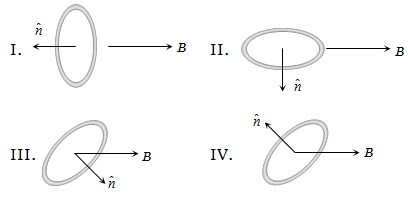(a) I > III > II > IV                               (b) I > II >III > IV
(c) I > IV > II > III                               (d) III > IV > I > II

Concept Questions :-

Current carrying loop : Force and torque
High Yielding Test Series + Question Bank - NEET 2020

Difficulty Level:

A metallic block carrying current I is subjected to a uniform magnetic induction $\stackrel{⇁}{B}$ as shown in the figure. The moving charges experience a force given $\stackrel{⇁}{F}$ by ........... which results in the lowering of the potential of the face ........ Assume the speed of the carriers to be v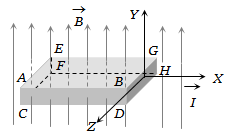(a) $eVB\stackrel{^}{k}$ , ABCD                                (b) $eVB\stackrel{^}{k}$ , EFGH

(c) $-eVB\stackrel{^}{k}$ , ABCD                              (d) $-eVB\stackrel{^}{k}$ , EFGH

Concept Questions :-

Lorentz force
High Yielding Test Series + Question Bank - NEET 2020

Difficulty Level:

Two insulated rings, one of slightly smaller diameter than the other are suspended along their common diameter as shown. Initially the planes of the rings are mutually perpendicular. When a steady current is set up in each of them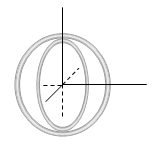(a) The two rings rotate into a common plane

(b) The inner ring oscillates about its initial position

(c) The inner ring stays stationary while the outer one moves into the plane of the inner ring

(d) The outer ring stays stationary while the inner one moves into the plane of the outer ring

Concept Questions :-

Magnetic field due to various cases

Difficulty Level:

Two particles each of mass m and charge q are attached to the two ends of a light rigid rod of length 2R. The rod is rotated at constant angular speed about a perpendicular axis passing through its centre. The ratio of the magnitudes of the magnetic moment of the system and its angular momentum about the centre of the rod is:

1. $\frac{q}{2m}$

2. $\frac{q}{m}$

3. $\frac{2q}{m}$

4. $\frac{q}{\mathrm{\pi m}}$

Concept Questions :-

Magnetic moment
High Yielding Test Series + Question Bank - NEET 2020

Difficulty Level:

A ring of radius R, made of an insulating material carries a charge Q uniformly distributed on it. If the ring rotates about the axis passing through its center and normal to the plane of the ring with constant angular speed $\omega$, then the magnitude of the magnetic moment of the ring is:

1. $Q\omega {R}^{2}$                       2. $\frac{1}{2}Q\omega {R}^{2}$

3. $Q{\omega }^{2}R$                       4. $\frac{1}{2}Q{\omega }^{2}R$

Concept Questions :-

Magnetic moment
High Yielding Test Series + Question Bank - NEET 2020

Difficulty Level:

Three long, straight and parallel wires carrying currents are arranged as shown in the figure. The wire C which carries a current of 5.0 amp is so placed that it experiences no force. The distance of wire C from wire D is then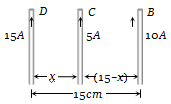(a) 9 cm                                          (b) 7 cm

(c) 5 cm                                           (d) 3 cm

Concept Questions :-

Force between current carrying wires
High Yielding Test Series + Question Bank - NEET 2020

Difficulty Level:

If m is the magnetic moment and B is the magnetic field, then the torque is given by

(a) $\stackrel{\to }{m}.\stackrel{\to }{B}$                       (b) $\frac{|\stackrel{\to }{m}|}{|\stackrel{\to }{B}|}$

(c) $\stackrel{\to }{m}×\stackrel{\to }{B}$                      (d) $|\stackrel{\to }{m}|.|\stackrel{\to }{B}|$

Concept Questions :-

Magnetic moment

Difficulty Level:

What is the net force on the square coil ?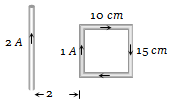(a) $25×{10}^{-7}N$ moving towards wire

(b) $25×{10}^{-7}N$ moving away from wire

(c) $35×{10}^{-7}N$ moving towards wire

(d) $35×{10}^{-7}N$ moving away from wire

High Yielding Test Series + Question Bank - NEET 2020

Difficulty Level:

Two long parallel copper wires carry currents of 5A each in opposite directions. If the wires are separated by a distance of 0.5 m, then the force between the two wires is :

(a) ${10}^{-5}N,$ attractive                (b) ${10}^{-5}N,$ repulsive

(c) $2×{10}^{-5}N,$ attractive           (d) $2×{10}^{-5}N,$ repulsive

Concept Questions :-

Force between current carrying wires
High Yielding Test Series + Question Bank - NEET 2020

Difficulty Level:

A long wire A carries a current of 10 amp. Another long wire B, Which is parallel to A and separated by 0.1m from A, carries a current of 5 amp, in the opposite direction to that in A. what is the magnitude and nature of the force experienced per unit length of B $\left({\mu }_{0}=4\mathrm{\pi }×{10}^{-7}weber/amp-\mathrm{m}\right)$

(a) Repulsive force of ${10}^{-4}N/m$

(b) Attractive force of ${10}^{-4}N/m$

(c) Repulsive force of $2\mathrm{\pi }×{10}^{-5}\mathrm{N}/\mathrm{m}$

(d) Attractive force of $2\mathrm{\pi }×{10}^{-5}\mathrm{N}/\mathrm{m}$

Concept Questions :-

Force between current carrying wires